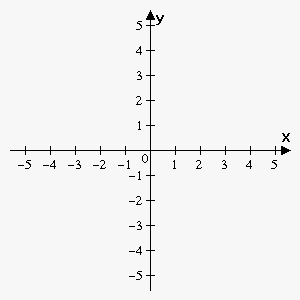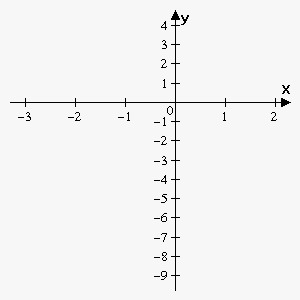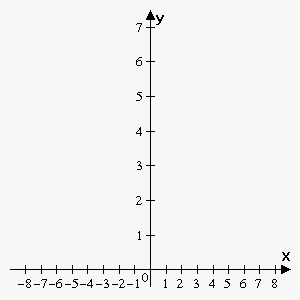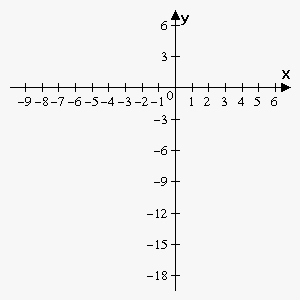# Steps for Sketching the Graph of the Function

## Verwandter Rechner: Grafikrechner

Suppose we are given continuous on ${\left[{a},{b}\right]}$ function $y={f{{\left({x}\right)}}}$ that is twice differentiable, except points where the derivative $f{'}\left({x}\right)$ doesn't exist or has infinite value.

To sketch the graph of a function, we need to perform the following:

1. Determine, whether function is obtained by transforming a simpler function, and perform necessary steps for this simpler function.
2. Determine, whether function is even, odd or periodic. This allows to draw graph of the function on some subinterval and then just reflect the result.
3. Find y-intercept (point ${f{{\left({0}\right)}}}$).
4. Find x-intercepts (points where ${f{{\left({x}\right)}}}={0}$).
5. Find what asymptotes does function have, if any.
6. Calculate first derivative and find points where ${f{'}}{\left({x}\right)}={0}$ or ${f{'}}{\left({x}\right)}$ doesn't exist, in other words find stationary points.
7. Use a test (the first derivative or the second derivative) to classify stationary points.
8. Find intervals where the function is increasing $\left({f{'}}{\left({x}\right)}>{0}\right)$ and where it is decreasing $\left({f{'}}{\left({x}\right)}<{0}\right)$.
9. Find points where ${f{''}}{\left({x}\right)}={0}$ or ${f{''}}{\left({x}\right)}$ doesn't exist and test whether these points are points of inflection.
10. Find where function is concave up $\left({f{''}}{\left({x}\right)}>{0}\right)$ and where it is concave down $\left({f{''}}{\left({x}\right)}<{0}\right)$.
11. After you've found "important" points calculate corresponding values of function at these points.
12. Add "control" points (some arbitrary points), if needed.
13. Draw "important" and "control" points and connect them by lines taking into account found behavior of the function.
14. If function is even, odd or periodic then perform corresponding reflection.
15. If function is obtained by transforming simpler function, perform corresponding shift, compressing/stretching.

It is often convenient to draw all points, you've found, in the table.

Example 1. Sketch graph of the function ${f{{\left({x}\right)}}}=\frac{{1}}{{{x}-{1}}}$.

Since ${f{{\left({x}\right)}}}={g{{\left({x}-{1}\right)}}}$ where ${g{{\left({x}\right)}}}=\frac{{1}}{{x}}$ then we study function ${g{{\left({x}\right)}}}=\frac{{1}}{{x}}$ and then shift graph one unit to the right.

Function is odd because ${g{{\left({x}\right)}}}=-{g{{\left(-{x}\right)}}}$, so we consider only interval ${\left[{0},\infty\right)}$ and then just reflect result about the origin. Function is not periodic.

There is no y-intercept because ${g{{\left({0}\right)}}}$ is undefined.

There is no x-intercept because there are no points where ${g{{\left({x}\right)}}}=\frac{{1}}{{x}}={0}$.

Since $\lim_{{{x}\to{{0}}^{+}}}\frac{{1}}{{x}}=+\infty$ then ${x}={0}$ is vertical asymptote.

Since $\lim_{{{x}\to\infty}}\frac{{1}}{{x}}={0}$ then ${y}={0}$ is horizontal asymptote.

We have that ${g{'}}{\left({x}\right)}=-\frac{{1}}{{{x}}^{{2}}}$. There are no points where derivative equals 0. Point ${x}={0}$ is where derivative doesn't exist.

Since ${g{'}}{\left({x}\right)}=-\frac{{1}}{{{x}}^{{2}}}<{0}$ for all ${x}$ then function is decreasing on ${\left({0},+\infty\right)}$ and there are no extrema according to First Derivative Test.

Second derivative is ${g{''}}{\left({x}\right)}=\frac{{2}}{{{x}}^{{3}}}$. There are no points where ${g{''}}{\left({x}\right)}={0}$ and second derivative doesn't exist when ${x}={0}$.Since second derivative is positive for ${x}>{0}$ then function is concave upward on ${\left({0},\infty\right)}$.

Point ${x}={0}$ is not point of inflection because ${x}={0}$ is not in the domain of the function.

So, we actually don't have important points, but when ${x}$ approaches 0 from the right function grows without a bound and we can sketch function, taking into account that functions is decreasing and concave upward on ${\left({0},+\infty\right)}$. For better graph take a couple of control points: ${\left({1},{1}\right)},{\left({1},\frac{{1}}{{2}}\right)},{\left({4},\frac{{1}}{{4}}\right)}$.

Since function is odd, reflect it about origin.

Shift the function 1 unit to the right.

Example 2. Sketch graph of the function ${f{{\left({x}\right)}}}={{\left({x}+{2}\right)}}^{{2}}{{\left({x}-{1}\right)}}^{{3}}$.

There is no simpler function that initial function is obtained from.

Function is neither even nor odd and not periodic.

${f{{\left({0}\right)}}}={{\left({0}+{2}\right)}}^{{2}}{{\left({0}-{1}\right)}}^{{3}}=-{4}$, so y-intercept is -4.

x-intercepts are points where ${f{{\left({x}\right)}}}={0}$: ${{\left({x}+{2}\right)}}^{{2}}{{\left({x}-{1}\right)}}^{{3}}={0}$ which gives ${x}=-{2}$ and ${x}={1}$.

Since function is continuous everywhere, $\lim_{{{x}\to-\infty}}{f{{\left({x}\right)}}}=-\infty$ and $\lim_{{{x}\to\infty}}{f{{\left({x}\right)}}}=\infty$ then there are no asymptotes.

We have that ${f{'}}{\left({x}\right)}={2}{\left({x}+{2}\right)}{{\left({x}-{1}\right)}}^{{3}}+{3}{{\left({x}+{2}\right)}}^{{2}}{{\left({x}-{1}\right)}}^{{2}}={\left({x}+{2}\right)}{{\left({x}-{1}\right)}}^{{2}}{\left({2}{x}-{2}+{3}{x}+{6}\right)}=$

$={\left({x}+{2}\right)}{{\left({x}-{1}\right)}}^{{2}}{\left({5}{x}+{4}\right)}$.

So, derivative equals 0 when ${x}=-{2},{x}={1},{x}=-\frac{{4}}{{5}}$.

According to method of intervals number line is divided by stationary points on four intervals.

First interval is ${\left(-\infty,-{2}\right)}$: taking any point from this interval, for example ${x}=-{3}$ we obtain that ${f{'}}{\left(-{3}\right)}={\left(-{3}+{2}\right)}{{\left(-{3}-{1}\right)}}^{{2}}{\left({5}\cdot{\left(-{3}\right)}+{4}\right)}>{0}$, so ${f{'}}{\left({x}\right)}>{0}$ on this interval.

Second interval is ${\left(-{2},-\frac{{4}}{{5}}\right)}$: taking any point from this interval, for example ${x}=-{1}$ we obtain that ${f{'}}{\left(-{1}\right)}={\left(-{1}+{2}\right)}{{\left(-{1}-{1}\right)}}^{{2}}{\left({5}\cdot{\left(-{1}\right)}+{4}\right)}<{0}$, so ${f{'}}{\left({x}\right)}<{0}$ on this interval.

Third interval is ${\left(-\frac{{4}}{{5}},{1}\right)}$: taking any point from this interval, for example ${x}={0}$ we obtain that ${f{'}}{\left({0}\right)}={\left({0}+{2}\right)}{{\left({0}-{1}\right)}}^{{2}}{\left({5}\cdot{\left({0}\right)}+{4}\right)}>{0}$, so ${f{'}}{\left({x}\right)}>{0}$ on this interval.

Fourth interval is ${\left({1},\infty\right)}$: taking any point from this interval, for example ${x}={3}$ we obtain that ${f{'}}{\left({3}\right)}={\left({3}+{2}\right)}{{\left({3}-{1}\right)}}^{{2}}{\left({5}\cdot{3}+{4}\right)}>{0}$, so ${f{'}}{\left({x}\right)}>{0}$ on this interval.

According to First Derivative Test ${x}=-{2}$ is local maximum, ${x}=-\frac{{4}}{{5}}$ is local minimum and ${x}={1}$ is not an extremum.Second derivative is ${f{''}}{\left({x}\right)}={2}{\left({x}-{1}\right)}{\left({10}{{x}}^{{2}}+{16}{x}+{1}\right)}$.

Roots of the equation ${10}{{x}}^{{2}}+{16}{x}+{1}={0}$ are ${x}=\frac{{-{8}+{3}\sqrt{{{6}}}}}{{10}}\approx-{0.065}$ and ${x}=\frac{{-{8}-{3}\sqrt{{{6}}}}}{{10}}\approx-{1.535}$.

So, points where second derivative equals 0 are ${x}={1},{x}=-{0.065},{x}=-{1.535}$.

Clearly they are all points of inflections because second derivative changes sign at these points.

${f{''}}{\left({x}\right)}>{0}$ on ${\left(-{1.535},-{0.065}\right)}\cup{\left({1},\infty\right)}$ and ${f{''}}{\left({x}\right)}<{0}$ on ${\left(-\infty,-{1.535}\right)}\cup{\left(-{0.0065},{1}\right)}$.

So we have following "important" points:

 ${x}$ -2 -1.535 $-\frac{{4}}{{5}}$ -0.065 0 1 ${f{{\left({x}\right)}}}$ 0 -3.52241 -8.3981 -4.052 -4 0 type maximum, x-intercept inflection minimum inflection y-intercept inflection, x-intercept

Function is increasing on ${\left(-\infty,-{2}\right)}\cup{\left(-\frac{{4}}{{5}},\infty\right)}$ and decreasing on ${\left(-{2},-\frac{{4}}{{5}}\right)}$.

Function is concave upward on ${\left(-{1.535},-{0.065}\right)}\cup{\left({1},\infty\right)}$ and concave downward on ${\left(-\infty,-{1.535}\right)}\cup{\left(-{0.0065},{1}\right)}$.

Note that points ${\left(-{0.065},-{4.052}\right)}$ and ${\left({0},-{4}\right)}$ are very close, so they are almost indistinguishable on graph.

Example 3. Sketch graph of the function ${f{{\left({x}\right)}}}=\frac{{{{x}}^{{2}}-{5}{x}+{6}}}{{{{x}}^{{2}}+{1}}}$.

There is no simpler function that initial function is obtained from.

Function is neither even nor odd and not periodic.

${f{{\left({0}\right)}}}=\frac{{{{0}}^{{2}}-{5}\cdot{0}+{6}}}{{{{0}}^{{2}}+{1}}}={6}$, so y-intercept is 6.

x-intercepts are points where ${f{{\left({x}\right)}}}={0}$: ${\left({{x}}^{{2}}-{5}{x}+{6}\right)}={\left({x}-{2}\right)}{\left({x}-{3}\right)}={0}$ which gives ${x}={2}$ and ${x}={3}$.

Since function is continuous everywhere, $\lim_{{{x}\to\infty}}\frac{{{{x}}^{{2}}-{5}{x}+{6}}}{{{{x}}^{{2}}+{1}}}={1}$ then there is only one asymptote: horizontal asymptote ${y}={1}$.

We have that ${f{'}}{\left({x}\right)}=\frac{{{\left({{x}}^{{2}}-{5}{x}+{6}\right)}'{\left({{x}}^{{2}}+{1}\right)}-{\left({{x}}^{{2}}-{5}{x}+{6}\right)}{\left({{x}}^{{2}}+{1}\right)}'}}{{{\left({{x}}^{{2}}+{1}\right)}}^{{2}}}={5}\frac{{{{x}}^{{2}}-{2}{x}-{1}}}{{{\left({{x}}^{{2}}+{1}\right)}}^{{2}}}$.

Roots of the equation ${{x}}^{{2}}-{2}{x}-{1}={0}$ are ${x}={1}+\sqrt{{{2}}}\approx{2.41}$ and ${x}={1}-\sqrt{{{2}}}\approx-{0.41}$.

So, derivative equals 0 when ${x}=-{0.41},{x}={2.41}$.

According to method of intervals number line is divided by stationary points on three intervals.

First interval is ${\left(-\infty,-{0.41}\right)}$: ${f{'}}{\left({x}\right)}>{0}$ on this interval.

Second interval is ${\left(-{0.41},{2.41}\right)}$:${f{'}}{\left({x}\right)}<{0}$ on this interval.

Third interval is ${\left({2.41},\infty\right)}$: ${f{'}}{\left({x}\right)}>{0}$ on this interval.

According to First Derivative Test ${x}=-{0.41}$ is local maximum, ${x}={2.41}$ is local minimum.Second derivative is ${f{''}}{\left({x}\right)}=-{10}\frac{{{\left({x}+{1}\right)}{\left({{x}}^{{2}}-{4}{x}+{1}\right)}}}{{{\left({{x}}^{{2}}+{1}\right)}}^{{3}}}$.

Roots of the equation ${{x}}^{{2}}-{4}{x}+{1}={0}$ are ${x}={2}-\sqrt{{{3}}}\approx{0.27}$ and ${x}={2}+\sqrt{{{3}}}\approx{3.73}$.

So, points where second derivative equals 0 are ${x}=-{1},{x}={0.27},{x}={3.73}$.

Clearly they are all points of inflections because second derivative changes sign at these points.

${f{''}}{\left({x}\right)}>{0}$ on ${\left(-\infty,-{1}\right)}\cup{\left({0.27},{3.73}\right)}$ and ${f{''}}{\left({x}\right)}<{0}$ on ${\left(-{1},{0.27}\right)}\cup{\left({3.73},\infty\right)}$.

So we have following "important" points:

 ${x}$ -1 -0.41 0 0.27 2 2.41 3 3.73 ${f{{\left({x}\right)}}}$ 6 7.0354 6 4.402 0 -0.0355 0 0.0847 type inflection maximum y-intercept inflection x-intercept minimum x-intercept inflection

Function is increasing on ${\left(-\infty,-{0.41}\right)}\cup{\left({2.41},\infty\right)}$ and decreasing on ${\left(-{0.41},{2.41}\right)}$.

Function is concave upward on ${\left(-\infty,-{1}\right)}\cup{\left({0.27},{3.73}\right)}$ and concave downward on ${\left(-{1},{0.27}\right)}\cup{\left({3.73},\infty\right)}$.

Horizontal asymptote is ${y}={1}$.

Example 4. Sketch graph of the function ${f{{\left({x}\right)}}}=\frac{{{\left({x}-{1}\right)}}^{{3}}}{{{\left({x}+{1}\right)}}^{{2}}}$.

There is no simpler function that initial function is obtained from.

Function is neither even nor odd and not periodic.

${f{{\left({0}\right)}}}=\frac{{{\left({0}-{1}\right)}}^{{3}}}{{{\left({0}+{1}\right)}}^{{2}}}=-{1}$, so y-intercept is -1.

x-intercepts are points where ${f{{\left({x}\right)}}}={0}$: ${{\left({x}-{1}\right)}}^{{3}}={0}$ which gives ${x}={1}$.

Vertical asymptote is ${x}=-{1}$ because $\lim_{{{x}\to-{{1}}^{+}}}\frac{{{\left({x}-{1}\right)}}^{{3}}}{{{\left({x}+{1}\right)}}^{{2}}}=-\infty$.

There are no horizontal asymptotes because $\lim_{{{x}\to\infty}}\frac{{{\left({x}-{1}\right)}}^{{3}}}{{{\left({x}+{1}\right)}}^{{2}}}=\infty$ and $\lim_{{{x}\to-\infty}}\frac{{{\left({x}-{1}\right)}}^{{3}}}{{{\left({x}+{1}\right)}}^{{2}}}=-\infty$.

Since $\lim_{{{x}\to\infty}}\frac{{{f{{\left({x}\right)}}}}}{{x}}=\lim_{{{x}\to\infty}}\frac{{{{x}}^{{3}}-{3}{{x}}^{{2}}+{3}{x}-{1}}}{{{{x}}^{{3}}+{2}{{x}}^{{2}}+{x}}}={1}$ then ${m}={1}$. Now $\lim_{{{x}\to\infty}}{\left({f{{\left({x}\right)}}}-{m}{x}\right)}=\frac{{{{x}}^{{3}}-{3}{{x}}^{{2}}+{3}{x}-{1}}}{{{{x}}^{{2}}+{2}{x}+{1}}}-{x}=\lim_{{{x}\to\infty}}\frac{{-{5}{{x}}^{{2}}+{2}{x}-{1}}}{{{{x}}^{{2}}-{2}{x}+{1}}}=-{5}$.

So, there is slant asymptote ${y}={x}-{5}$.

We have that ${f{'}}{\left({x}\right)}=\frac{{{\left({{\left({x}-{1}\right)}}^{{3}}\right)}'{{\left({x}+{1}\right)}}^{{2}}-{{\left({x}-{1}\right)}}^{{3}}{\left({{\left({x}+{1}\right)}}^{{2}}\right)}'}}{{{\left({x}+{1}\right)}}^{{4}}}=\frac{{{{\left({x}-{1}\right)}}^{{2}}{\left({x}+{5}\right)}}}{{{\left({x}+{1}\right)}}^{{3}}}$.

Derivative equals 0 when ${x}={1},{x}=-{5}$ and doesn't exist when ${x}=-{1}$.

Since ${{\left({x}-{1}\right)}}^{{2}}\ge{0}$ then these factor doesn't influence sign of derivative.

Thus ${f{'}}{\left({x}\right)}>{0}$ when ${x}\in{\left(-\infty,-{5}\right)}\cup{\left(-{1},\infty\right)}$ and ${f{'}}{\left({x}\right)}<{0}$ when ${x}\in{\left(-{5},-{1}\right)}$.

According to First Derivative Test ${x}=-{5}$ is local maximum and ${x}={1}$ is not an extremum.Point ${x}=-{1}$ is not in the domain of function, so clearly not an extremum.

Second derivative is ${f{''}}{\left({x}\right)}={24}\frac{{{x}-{1}}}{{{\left({x}+{1}\right)}}^{{4}}}$.

So, point where second derivative equals 0 is ${x}={1}$.

Clearly it is inflection point because second derivative changes sign at this point.

${f{''}}{\left({x}\right)}>{0}$ when ${x}>{1}$ and ${f{''}}{\left({x}\right)}<{0}$ when ${x}<{1}$.

Let's add a couple of control points:

${x}={3}$, ${f{{\left({3}\right)}}}={0.5}$.

${x}=-{8}$, ${f{{\left(-{8}\right)}}}\approx-{14.88}$.

So, we have following points:

 ${x}$ -8 -5 0 1 3 ${f{{\left({x}\right)}}}$ -14.88 -13.5 -1 0 0.5 type control maximum y-intercept inflection control

Function is increasing on ${\left(-\infty,-{5}\right)}\cup{\left(-{1},\infty\right)}$ and decreasing on ${\left(-{5},-{1}\right)}$.

Function is concave upward on ${\left({1},\infty\right)}$ and concave downward on ${\left(-\infty,{1}\right)}$.

Vertical asymptote is ${x}=-{1}$ and slant asymptote is ${y}={x}-{5}$.